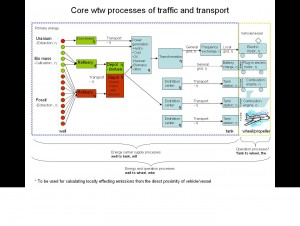# Metoder

## General format

• The decimal point is denoted with a period, e.g. 1.234
• Scientific notation is used to write numbers that are too big or too small to be conveniently written in decimal form. In scientific notation all numbers are written in the form of a*10b (a times ten raised to the power of b). Because superscripted exponents cannot always be conveniently displayed, the letter E is used to represent times ten raised to the power of, e.g. 2*103 is written as 2E3. Examples:
• 12E3 is 1200 with two significant figures
• 12.00E3 is 1200 with four significant figures
• 1.23E0 is 1.23
• 1.23E-1 is 0.123
• 1.23E-3 is 0.00123

## Rounding and significant figures

### Rounding of measurements to significant figures

Most numerical data handled by the system (user input, database values, etc) represent approximate measurements and it is important that reported numbers do not appear to be more accurate than they really are. This will require the system to implement a proper set of rules for rounding, and for this purpose the concept of significant figures has been adopted. In short this means that numbers are rounded to the last figure that has accuracy. If distance would be measured with a measure tape used for carpentry, any measurement smaller than a millimeter would be of very low accuracy due to the precision of the device, and followingly a measurement of 4.5678 meters would be rounded to 4.568 meters (the fourth digit being the last digit of accuracy). Examples:

• 1.234 rounded to two significant figures is 1.2
• 7.5678 rounded to one significant figure is 8
• 1234 rounded to two significant figures is 1200 or 12E2 depending on whether trailing zeroes are considered to be significant or not. In NTMCalc this differs between input and output data, please review the sections below for more detail on this.